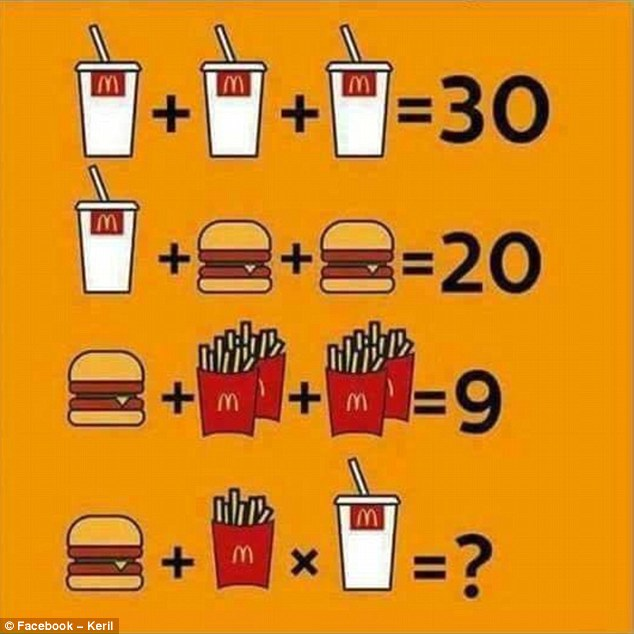## Can You Solve This?

Posted By on January 27, 2017

## Here’s a brainteaser, can you solve it?HOW TO SOLVE IT

To work out the answer, you firstly need to understand the cost of each product.

From the first line, you can tell that each drink is worth 10, while on the second line you can decipher that a burger is worth five.

On the third line – remembering that there is a total of four packet of chips – you can see that each packet of chips is worth one.

Finally, applying the rule that multiplication comes before addition, you can multiply the chips by the drink, totaling 10, before adding the burger, giving the answer 15.

10+10+10 = 30

10+5+5 = 20

5+2+2 = 9

5+1×10 = 15